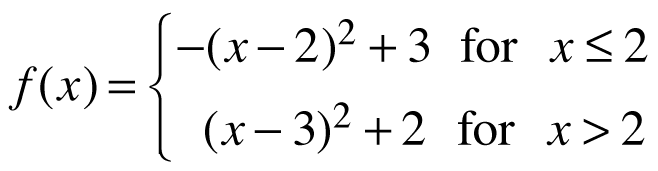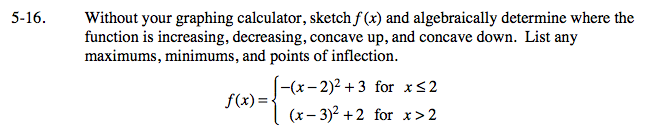### Home > CALC > Chapter 5 > Lesson 5.1.2 > Problem5-16

5-16.

Without your graphing calculator, sketch f(x) and algebraically determine where the function is increasing, decreasing, concave up, and concave down. List any maximums, minimums, and points of inflection. Homework Help ✎A function is increasing where its first derivative > 0 and decreasing where its first derivative is < 0.
Find these intervals algebraically.

A function is concave up when its second derivative is > 0 and concave down when its second derivative is < 0.
Find these intervals algebraically.

Notice that x = 2 is a boundary point. Even though f '(2) ≠ 0 and f ''(2) ≠ 0, interesting changes in slope and concavity can still happen at x = 2.